ASCII data## ASCII data

بازدید : 1235 چهارشنبه 10 دي 1393

## ASCII or Text Data and grads

Input ASCII data

grads does not directly handle data in ASCII format -- there is no such thing as 'dtype ascii'. ASCII data must be converted to into one of the binary data formats that are handled by grads. There are many ways to do this with a variety of programming/scripting languages and tools. Below is a very simple example of how you could do it using a grads script.

Suppose you have an ascii file that contains a column of 100 numbers:

```
18864

20844

27388

24124
36884

...

39836

```

Here is a how you would convert that to a binary file using grads. You must have a file open for this to work, any file will do.

```
'open dummy.ctl'

file = 'my_ascii_file.txt'

datafile = 'my_binary_file.dat'

'set gxout fwrite'

'!/bin/rm -f 'datafile

'set fwrite -ap 'datafile
while (1)

line2 = sublin(res,2)

line1 = sublin(res,1)

val = subwrd(line2,1)

rc1 = subwrd(line1,1)
if (rc1); break; endif
'd 'val

'disable fwrite'

endwhile

rc = close(file)

```
The descriptor file would look like this, assuming you wanted to map your data into the X dimension:
```

dset ^my_binary_file.dat

title Sample of ASCII data converted to binary

undef -9.99e8
xdef 100 linear 1 1

tdef 1 linear 01jan0001 1dy

ydef 1 linear 1 1
zdef 1 linear 1 1
vars 1

endvars

a 0 99 ascii variable

```

Output Gridded Data in ASCII Format

1. GrADS can create ascii output for any display with the 'set gxout print' option. The formatting of the output is controlled with the 'set prnopts' command. The output is printed to the command window, but it is also stored in the internal variable 'result' inside a script, where it can be easily output to a file. Below is a simple script example to write formatted ascii output to a file. Note that the choice of writing 72 numbers per line is based on the X dimension of the grid being displayed.

```
'open /data/grib/model/model.ctl'

'set x 1 72'

'set gxout print'

outfile = 'my_ascii_file.txt'

rc = write(outfile,result)

'set prnopts %7.3f 72 1'

'd ts'

```

The beginning of the file my_ascii_file.txt looks like this:

```
Printing Grid -- 3312 Values -- Undef = -9.99e+08

258.493 258.493 258.493 258.493 258.493 258.493 258.493 258.493 258.493 258.493 258.493 <etc.>

```

2. In the above example, note that the first line of the output file will contain some diagnostic information about the number of values written and the undef value. If you want to omit that line and write out just the ascii data, then use this slightly more complicated version of the script which skips over the first line of diagnostic info. Two other changes in this example are in the formatting (the output will be comma delimited for importing into a spreadsheet) and the displayed variable, which is now an expression instead of a variable name. It is not necessary to 'define' the expression before displaying it.

```
'open /data/grib/model/model.ctl'

'set x 1 72'

'!/bin/rm -f 'outfile

outfile='my_ascii_file.txt'

'd ts-273.15'

'set gxout print'
'set prnopts %g, 72 0'
i=1

if (line = ''); break; endif

while (1)
line = sublin(result,i)
if (i>1)

endwhile

rc = write(outfile,line,append)
endif

i=i+1

```

Now the beginning of the output file my_ascii_file.txt looks like this:

```-14.6567,-14.6567,-14.6567,-14.6567,-14.6567,-14.6567,-14.6567,-14.6567,-14.6567,<etc.>
```

3. If you want to write out the grid information along with data from more than one variable, then you must save the result from the display of each variable/expression (including longitude and latitude), parse each data point individually, construct the line of ascii output, and then write it out. Here is an example the writes out longitude, latitude, surface temperature, surface pressure, and precipitation:

```
'open /data/grib/model/model.ctl'

'set x 1 72'

'!/bin/rm -f 'outfile

outfile='my_ascii_file.txt'

'set prnopts 'fmt' 'numcols' 1'

'set gxout print'
fmt='%8.3f'
numcols=72

lat_data = result

'd lon'
lon_data = result
'd lat'
'd ts'

v3_data = result

v1_data = result
'd ps'
v2_data = result
'd p'
i=1

lats  = sublin(lat_data,i)

while (1)
lons  = sublin(lon_data,i)

line3 = sublin(v3_data,i)

line1 = sublin(v1_data,i)
line2 = sublin(v2_data,i)

if (lons='' | lats='' | line1='' | line2='' | line3=''); break; endif

if (i>1)
j=1
while (j<=numcols)
str = subwrd(lons,j); lon = math_format(fmt,str)

str = subwrd(line2,j); v2 = math_format(fmt,str)

str = subwrd(lats,j); lat = math_format(fmt,str)
str = subwrd(line1,j); v1 = math_format(fmt,str)

endwhile

str = subwrd(line3,j); v3 = math_format(fmt,str)
record = lon' 'lat' 'v1' 'v2' 'v3
rc = write(outfile,record,append)
j=j+1
endif
i=i+1

endwhile

```

Now the head of the output file my_ascii_file.txt looks like this:

```
0.000  -90.000  258.493  669.911    0.000

5.000  -90.000  258.493  669.911    0.000

15.000  -90.000  258.493  669.911    0.000

10.000  -90.000  258.493  669.911    0.000
20.000  -90.000  258.493  669.911    0.000

35.000  -90.000  258.493  669.911    0.000

25.000  -90.000  258.493  669.911    0.000
30.000  -90.000  258.493  669.911    0.000

40.000  -90.000  258.493  669.911    0.000

```
مطالب مرتبط
ارسال نظر برای این مطلب

نام
ایمیل (منتشر نمی‌شود) (لازم)
وبسایت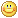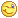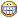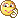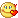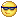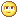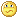نظر خصوصی
مشخصات شما ذخیره شود ؟ [حذف مشخصات] [شکلک ها]کد امنیتی
اطلاعات کاربری
 نام کاربری : رمز عبور :
• فراموشی رمز عبور؟
• لینک دوستان
آرشیو
آمار سایت
• کل مطالب : 155
• کل نظرات : 0
• افراد آنلاین : 1
• تعداد اعضا : 4
• آی پی امروز : 5
• آی پی دیروز : 10
• بازدید امروز : 40
• باردید دیروز : 67
• گوگل امروز : 0
• گوگل دیروز : 0
• بازدید هفته : 522
• بازدید ماه : 522
• بازدید سال : 22,455
• بازدید کلی : 143,507
• کدهای اختصاصی